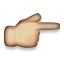# I don't understand how this var questionsLeft = "[" + questions +" questions left]" works.

The part that i don't understand is " + questions +" . Why do you use ""? isn't it suppose print about just a word? And those plus signs before and after. I understand why you need + after, but i don't understand why before.When used with strings, the + is a "concatenation operator". This means it joins strings together. The quote marks surround literal strings which will be used just as they are. So the whole thing builds a long string that starts with a bracket, then the number stored in "questions", then the phrase " questions left]".

So for example, if the variable "questions" was holding the number 3, after this statement runs the variable "questionsLeft" would contain this string[3 questions left]

i dont still understand that part its suppose to be questionleft=question +"questionleft" because we already gave the variable name a value of 3.And since the variable was given the value of 3, then this statement will convert it to a string and combine it the other words and symbols to display "[3 questions left]" on the screen.

Is this just a particularly hard bit of Javascript to get your head around or is it just very badly explained? I've watched this video about 6 times now and am none the wiser. AAARRGhhhh!!!!!The way I understand it....in {}.

var questions = 3;
{var is the named location; questions is the value}

var questionsLeft = " [" + questions + " questions left]";
{We need a new value (questionsLeft) for a placeholder to contain the information we want to see in the dialog box; " [" + questions + " questions left]"; second pair of quotes followed by a space gives us an actual space in the dialog box we see at the end; + of course is the string; questions is our number 3; third pair of quotes followed by a space, again actual space we see in the result; questions left are the actual words in the dialog box}